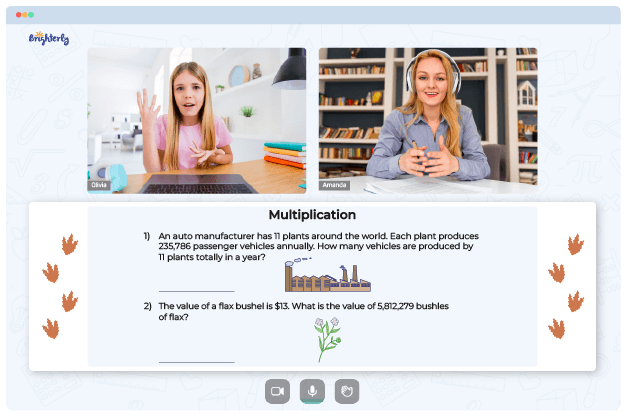# Multiplication And Division Worksheets

Since it is common for some kids to have complications when trying to study and understand math, educators developed math worksheets multiplication and division. These resources break down the learning process into digestible chunks.

There are many types of worksheets, for example, a multiplying and dividing negative numbers worksheet. This article will open your eyes to the benefits of getting worksheets for your kids.

## All about Division and Multiplication Worksheets

Children must understand the relationship between division and multiplication, starting from knowing that both calculations are an inverse of the other. They can begin with multi digit division worksheets to gain more knowledge and develop more skills as they explore the relationship between both operations.

To understand multiplication and division, children must know how to count from 1 to 30 and understand the concept of adding and subtracting. As kids proceed in their grades, they will be exposed to different exercises that develop skills and give knowledge on how to solve multiplication and division problems.

The division and multiplication worksheets give children analytical capabilities to tackle these operations. The tool also helps them with a systematic approach to solving multiplication and division problems, making youngsters understand each concept and its connections well.

Math for Kids

Is Your Child Struggling With Math?
1:1 Online Math TutoringThe worksheets for practicing multiplication and division expose kids to various math tasks. Understanding the connection between multiplication and division paves the way for mastering other fundamental arithmetic operations. Practicing with worksheets often strengthens kids’ ability to solve problems, critical thinking, and memorization.

## Benefits of Multiplication Division Worksheets

It is expected that children grasp the concept of multiplication and division as they proceed to higher grades. Kids who have difficulties solving multiplication and division exercises can turn to the worksheets for help. Worksheets allow kids to understand the concept better than if they were in a classroom listening to a teacher’s explanation.

The multiplication and division worksheet allows children to work on various math problems around those concepts. The task can come as puzzles, word problems, quantitative reasoning, etc. These worksheets have been the way forward in helping kids understand some of the basic operations.### Multiplication And Division Worksheets PDF

Division And Multiplication Worksheets### Multiplication And Division Worksheets PDF

Multiplication And Division Worksheet### Multiplication And Division Worksheets PDF

Math Worksheets Multiplication And Division### Multiplication And Division Worksheets PDF

Multiplication Division Worksheets

Multiplication and division worksheets contain creative math games and fun activities. They help boost and stimulate kids’ brain power and ability to do well in math. The tool also improves their retention power and ability to recognize multiplication and division problems irrespective of the form they take.

Combination of two mathematical operations is one of many elements of the average math curriculum for grade school kids. Worksheets containing multiplication and division enable kids to master those elements and make intelligent, logical decisions as they grow older. The multiplying and dividing rational numbers worksheet prepares kids for challenges that require their calculative prowess.

### More Multiplication Worksheets

Need help with Multiplication?• Is your child finding it challenging to understand multiplication?
• An online tutor could be the answer.

Does your child need additional assistance with mastering multiplication lessons? Start learning with an online tutor.